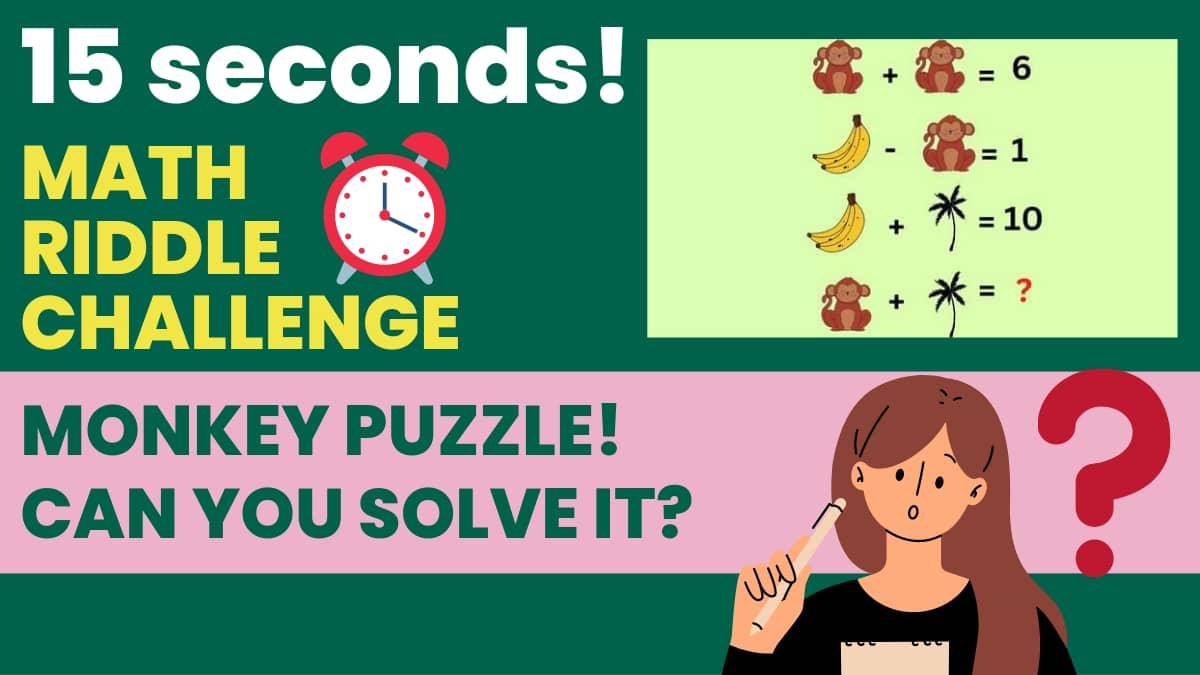# Math Riddles: Can you Solve This Monkey Puzzle In 15 Seconds? Test your IQ

Math Riddle Challenge: it's brain exercise time! Can you solve this puzzle In 15 Seconds? Test your IQ and sharpen your brain with this fruity math riddle.Math Riddles: Can you Solve This Monkey Puzzle In 15 Seconds? Test your IQ

Math Riddle Challenge: it's brain exercise time and we promise you that these simple math puzzles won't be as easy as you think ! So sit back, grab a piece of paper, pens or pencils and no calculators at all.

This is brain power time!

Can you solve this puzzle In 15 Seconds? Test your IQ and sharpen your brain with this fruity math riddle.

Look at the puzzle below carefully and you will find a math puzzle that only those with a high IQ will be able to answer. Are you one of them? Let's Find out!

Is it easy?

Remember  you have 15 seconds only!

All the best!

While you brainstorm here is some facts about a math puzzle

 Did you know ? Solving math riddles regularly can make you brighter and sharper by improving your cognitive functioning and enhancing your critical thinking and problem-solving abilities.

Hurry up! The clock's ticking!

Have you solved the brain teaser yet?

3…

2…

1…

### Time's up!

Could you solve it?

## First Equation:

Here we can see two monkeys(M) and their sum is 6.

So,

### M=3

Now we know that the value of Monkey is 3.

## Second equation

Here, we can see that the Monkey(M) subtracted by the Banana(B) is 1.

So,

### B=4

Now we know that the value of Banana is 4.

## Third Equation

This equation shows that the sum of Banana (B) and Tree(T) is equal to 10.

### T=6

Now we know that the value of Tree is 6.

## Final equation

Let's find the missing number!

What is the sum of Monkey (M) and Tree (T). Let's find out.

### So the answer is 9.

Great work!

You solved it!

ALSO CHECK OUT!

Math Riddles: Can you Solve This Tricky Teddy Puzzle In 30 Seconds? Check if you have a high IQ here!

Math Riddles: Solve This Chocolate Puzzle In 30 Seconds And Test Your IQ!

Math Riddles: Can You Solve This Heart Puzzle In 30 Seconds?

Math Riddles: Do You Have A High IQ? Solve These Figures and Find the Missing Number!

Get the latest General Knowledge and Current Affairs from all over India and world for all competitive exams.
खेलें हर किस्म के रोमांच से भरपूर गेम्स सिर्फ़ जागरण प्ले पर